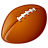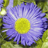# Problem doing a iteration with AddField and CalculateField -&gt; save Results in Table

2402
5
01-18-2012 05:15 AMNew Contributor
Hello i have a problem doing a iteration in my model:

The Model adds a field(called Leistung) in an attributetable and then calculates the value for this field. Afterwards i make a Ascii File(could also be another filetype) to save the Table with the added field. This calculated value is the Energy produced of a Photovoltaic Panel in one hour. Since i need to do this for more hours (and days and years) i want to have one file at the end where i have all the calculated values saved (and not one file for every hour). i dont know how to do this, can somebody help me or give me a hint?

in the picture i posted my model and the calculatfield statement.

btw. Im using ArcGis 9.3.

Florian
Tags (3)
5 RepliesOccasional Contributor
i didnt understand exactly what you want...

you'll always have only one field in your shapefile (called Leistung) to calculate this values, but in a lot of shapefiles... Is that right?

I think you should explain better what you want...New Contributor
Yes thats right, one field in many shapefiles. So what i need to do is to extract this field called Leistung from all the shapefiles and put it together in only one file, because i need to do further calculations with another program.

The aim is to avoid that i have thousands of shapefiles on my computer.

for example: if i do the calculation for one year, and the input values are given hourly i have 8760 output files, so i wanted one file with 8760 colums instead.

i hope this is more understandable.

I tried to draw a schematic maybe it helps to explain my problem!

[ATTACH=CONFIG]11289[/ATTACH]byMVP Honored Contributor
Yes thats right, one field in many shapefiles. So what i need to do is to extract this field called Leistung from all the shapefiles and put it together in only one file, because i need to do further calculations with another program.

The aim is to avoid that i have thousands of shapefiles on my computer.

for example: if i do the calculation for one year, and the input values are given hourly i have 8760 output files, so i wanted one file with 8760 colums instead.

i hope this is more understandable.

I tried to draw a schematic maybe it helps to explain my problem!

[ATTACH=CONFIG]11289[/ATTACH]

What license level do you have?  If you have an ArcInfo license you could instead Append all of the tables together and use the Pivot Table tool to create the columns.  The appended tables would each need a numeric field with field the name that you want.  The value in the field would be the number of the output column you want for each table (i.e., the first hour table would have a value of 1, the seccond would have a 2, etc.).  The only pain of the Pivot Table tool is that the number value is treated like a string, so 1 is followed by 10, by 100, by 1000, and then 11.  That could be overcome by adding 10000 to all numbers, which would properly sort out as FIELD10001, FIELD10002, etc.

I expect you will have problems getting a table to work with 8760 columns.  Shapefiles won't work with that many columns.  I'm not sure what the limit is for the various geodatabases.byMVP Honored Contributor
At ArcGIS 10 a file geodatabases can have 64K columns, but SDE is limited to 500 columns.  Anyway, I doubt you could get a table with over 8K of columns into any other format.  Also performance may suck as more columns are added to an fgdb based on this thread.  Be prepared for some serious performance issues arising with a process that tries to create over 8K fields.  The Pivot Table would probaly optimize some of the field creation process in memory rather than hitting the disk with each new Add Field geoprocessing operation.  So if you have ArcInfo, give that option some serious consideration.New Contributor
What license level do you have?  If you have an ArcInfo license you could instead Append all of the tables together and use the Pivot Table tool to create the columns.  The appended tables would each need a numeric field with field the name that you want.  The value in the field would be the number of the output column you want for each table (i.e., the first hour table would have a value of 1, the seccond would have a 2, etc.).  The only pain of the Pivot Table tool is that the number value is treated like a string, so 1 is followed by 10, by 100, by 1000, and then 11.  That could be overcome by adding 10000 to all numbers, which would properly sort out as FIELD10001, FIELD10002, etc.

I expect you will have problems getting a table to work with 8760 columns.  Shapefiles won't work with that many columns.  I'm not sure what the limit is for the various geodatabases.

I have a ArcView License, but maybe there is a chance (since i am a student and using the program for my diploma thesis) to get the ArcInfo license. Ok since i have read that there might be a problem working with so many columns, i thought that maybe im using the Arcgis program only to generate the files and then do further calculation with Matlab.

Since i am a beginner with Arcgis and i just started a month ago to work with this program i want to ask if its actually possible to realize the model i have in mind with Arcgis. My input data consists of a XY-Table with Photovoltaics (Zip-Code, PeakPower...), NC-Files of Solar Irradiance(hourly means) and NC-Files of Temperature(daily means). The goal of my model is to get the Energy produced within one year (and later on for more years, i have input data for 30 years) and validate this with energy-values submitted to the electricity-stock exchange.

My idea is to do this with Arcgis as far as possible. Since there is a lot of output data i need to minimize the number of files, thats why i want to put as much columns as possible in one file (any maybe do the calculation with matlab).

Here is a picture of my model up to now ( i splitted it in two models to test the functionality):

model1 does the spatial joins so i have a output class with the Photovoltaics (ZIP-Code ,Peak Power) joined with the Irradiance and the Temperature. model2 does the addField and CalculateField part and exports it to an Ascii File.

[ATTACH=CONFIG]11355[/ATTACH]

[ATTACH=CONFIG]11356[/ATTACH]

Do you think its possible to realize these steps for more input data?